# Geometry of shapesPage 2

#### WATCH ALL SLIDES

If a parallelogram has an area of A square units, a base of b units and a height of h units, then A = bh. (Do example 1 p. 530)

The area of a region is the sum of the areas of all its non-overlapping parts. (Do example 3 p. 531)

b

h

Slide 9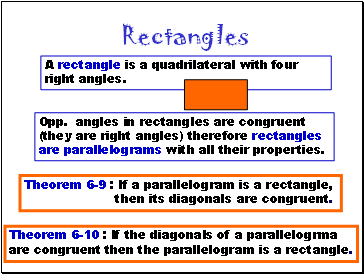## Rectangles

A rectangle is a quadrilateral with four right angles.

Theorem 6-9 : If a parallelogram is a rectangle,

then its diagonals are congruent.

Opp. angles in rectangles are congruent (they are right angles) therefore rectangles are parallelograms with all their properties.

Theorem 6-10 : If the diagonals of a parallelogrma

are congruent then the parallelogram is a rectangle.

Slide 10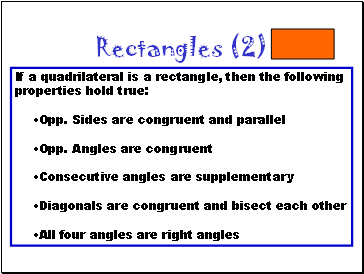Rectangles (2)

If a quadrilateral is a rectangle, then the following properties hold true:

Opp. Sides are congruent and parallel

Opp. Angles are congruent

Consecutive angles are supplementary

Diagonals are congruent and bisect each other

All four angles are right angles

Slide 11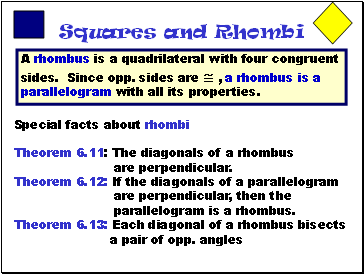## Squares and Rhombi

A rhombus is a quadrilateral with four congruent sides. Since opp. sides are  , a rhombus is a parallelogram with all its properties.

Theorem 6.11: The diagonals of a rhombus

are perpendicular.

Theorem 6.12: If the diagonals of a parallelogram are perpendicular, then the parallelogram is a rhombus.

Theorem 6.13: Each diagonal of a rhombus bisects

a pair of opp. angles

C

Slide 12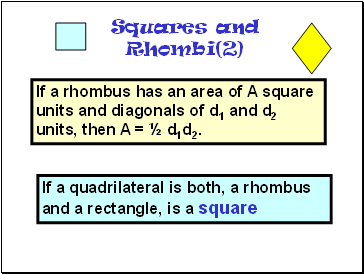Squares and Rhombi(2)

If a quadrilateral is both, a rhombus and a rectangle, is a square

If a rhombus has an area of A square units and diagonals of d1 and d2 units, then A = ½ d1d2.

Slide 13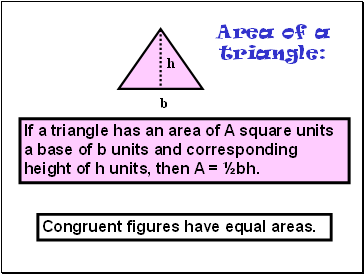## Area of a triangle

If a triangle has an area of A square units a base of b units and corresponding height of h units, then A = ½bh.

h

b

Congruent figures have equal areas.

Slide 14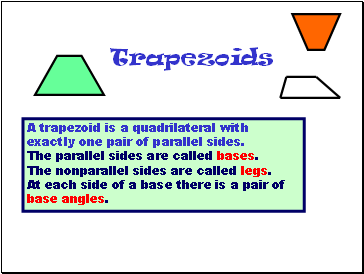## Trapezoids

A trapezoid is a quadrilateral with exactly one pair of parallel sides.

The parallel sides are called bases.

The nonparallel sides are called legs.

At each side of a base there is a pair of base angles.

C

Slide 15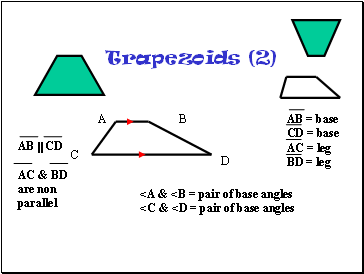Trapezoids (2)

C

A

C

D

B

AB = base

CD = base

AC = leg

BD = leg

AB  CD

AC & BD are non parallel

<A & <B = pair of base angles

Go to page:
1  2  3